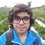Pre-RMO 2014/12

Let $ABCD$ be a convex quadrilateral with $\angle DAB = \angle BDC = 90^\circ$. Let the incircles of triangles $ABD$ and $BCD$ touch $BD$ at $P$ and $Q$, respectively, with $P$ lying in between $B$ and $Q$. If $AD = 999$ and $PQ = 200$ then what is the sum of the radii of the incircles of triangles $ABD$ and $BDC$?

This note is part of the set Pre-RMO 2014Note by Pranshu Gaba
5 years ago

This discussion board is a place to discuss our Daily Challenges and the math and science related to those challenges. Explanations are more than just a solution — they should explain the steps and thinking strategies that you used to obtain the solution. Comments should further the discussion of math and science.

When posting on Brilliant:

• Use the emojis to react to an explanation, whether you're congratulating a job well done , or just really confused .
• Ask specific questions about the challenge or the steps in somebody's explanation. Well-posed questions can add a lot to the discussion, but posting "I don't understand!" doesn't help anyone.
• Try to contribute something new to the discussion, whether it is an extension, generalization or other idea related to the challenge.
• Stay on topic — we're all here to learn more about math and science, not to hear about your favorite get-rich-quick scheme or current world events.

MarkdownAppears as
*italics* or _italics_ italics
**bold** or __bold__ bold

- bulleted
- list

• bulleted
• list

1. numbered
2. list

1. numbered
2. list
Note: you must add a full line of space before and after lists for them to show up correctly
paragraph 1

paragraph 2

paragraph 1

paragraph 2

> This is a quote
This is a quote
# I indented these lines
# 4 spaces, and now they show
# up as a code block.

print "hello world"
# I indented these lines
# 4 spaces, and now they show
# up as a code block.

print "hello world"
MathAppears as
Remember to wrap math in $$...$$ or $...$ to ensure proper formatting.
2 \times 3 $2 \times 3$
2^{34} $2^{34}$
a_{i-1} $a_{i-1}$
\frac{2}{3} $\frac{2}{3}$
\sqrt{2} $\sqrt{2}$
\sum_{i=1}^3 $\sum_{i=1}^3$
\sin \theta $\sin \theta$
\boxed{123} $\boxed{123}$

Sort by:

799

- 4 years, 8 months ago

Let $O_1$ and $O_2$ be the incenters of $\bigtriangleup DAB$ and $\bigtriangleup CDB$ respectively. Draw perpendicular from $O_1$ to sides $AD, DB$ and $AB$ at points $S, P$ and $E$ respectively. Draw perpendicular from $O_2$ to sides $CD$ and $DB$ at points $F$ and $Q$ respectively. Let $R$ and $r$ be the inradii of $\bigtriangleup DAB$ and $\bigtriangleup CDB$ respectively.

$SO_1EA$ and $FO_2QD$ are squares with side $r$ and $R$ respectively.

$DS=AD-R=999-r$

$DP=DQ+PQ=R+200$

Since $DP$ and $DS$ are tangents from the same point they are equal in length.

$\Rightarrow 999-r=R+200$

$\Rightarrow R+r=999-200= \boxed{799}$

- 5 years agoQ12

From the figure we can see that $SD = PD = PQ + QD = r_1 + 200$ and $AD = AS + SD =r_ 2 + SD = 999$.

$r_ 2 + r_1 + 200 = 999$

$r_1+ r_2 = \boxed{799}$

- 5 years ago

Why 799???

- 5 years ago

799

499.5 sqrt 2

- 5 years ago